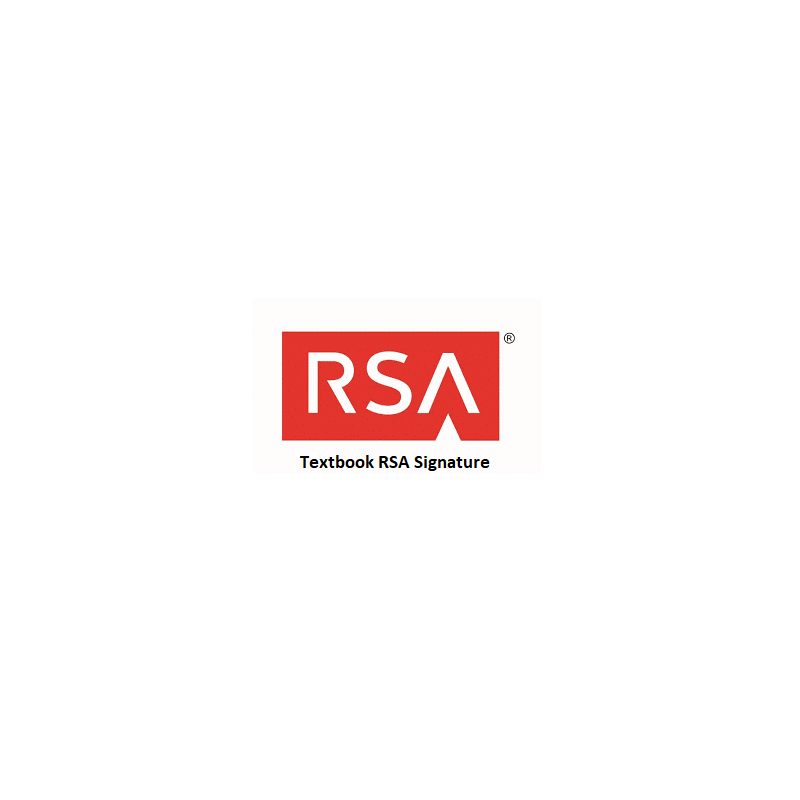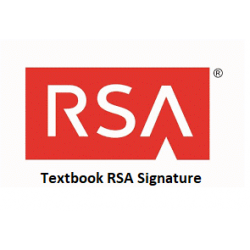•# RSA Textbook Signature

€2.00
Tax included

RSA textbook signature creation and verification.

Quantity

COMPLETED.

Task for the Student Alice is to generate RSA system parameters and send generated Public Key to the Mentor. Student must write down message containing current day and time in the format DDhhmm sign it by RSA textbook signature and send DDhhmm together with signature to the Mentor Bob.

After Student receives Mentor’s Public Key and signature on unknown message, he must verify this signature and recover the message. He must send recovered message to the Mentor.

Parameter values sent by Alice are included in brackets [ ] and must be entered in the corresponding input fields.

The following functions are used in the protocol:

>> genprime(14)

>> gcd(a,b)

>> mod(a,n)

>> eeuklid(e,ϕ)

>>mod_exp(a,x,n)

1. Compute RSA module n=pq by generating two prime numbers of 14 bit length using function

>> genprime(14)

Send numbers [p, q] for verification to the Mentor

1. If it is OK, find Euler’s totient function’s ϕ(n) value ϕ. Notice, that ϕ can be computed very effectively when p and q are given.

Send [ϕ] for verification to the Mentor

1. If it is OK, choose RSA exponent e satisfying condition gcd(e, ϕ)=1 and send computed RSA Public Key [n, e] for verification to the Mentor.

1. If it is OK, find RSA private key d using extended Euclidean algorithm

>> eeuklid(e,ϕ)

Send [d] for verification to the Mentor.

1. Sign message containing current day and time in the format DDhhmm computing signature SDT. Send [DDhhmm, SDT] to the Mentor for verification.

1. Mentor’s Public Key is nM=….. , eM=….. .  He sends You a signature SM=….. signed on some message M. Recover message M from the signature SM and send [M] to the Mentor for verification.
3099 Items